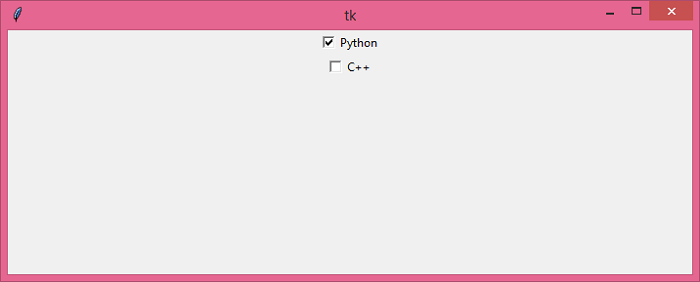# How to get the input from a Checkbox in Python Tkinter?

TkinterPythonGUI-Programming

A checkbox widget is an input widget that has two values, either True or False. A checkbox is useful in many applications where a particular value needs to be validated.

Let us suppose that we want to get the Input value from a checkbox such that if it is selected, then print the selected value. To print the value of the selected checkbox, we can use the get() method. It returns the input value of a particular widget.

## Example

# Import Tkinter library
from tkinter import *

# Create an instance of tkinter frame
win = Tk()

# Set the geometry of Tkinter frame
win.geometry("700x250")

# Define Function to print the input value
def display_input():
print("Input for Python:", var1.get())
print("Input for C++:", var2.get())

# Define empty variables
var1 = IntVar()
var2 = IntVar()

# Define a Checkbox
t1 = Checkbutton(win, text="Python", variable=var1, onvalue=1, offvalue=0, command=display_input)
t1.pack()
t2 = Checkbutton(win, text="C++", variable=var2, onvalue=1, offvalue=0, command=display_input)
t2.pack()

win.mainloop()

## Output

Executing the above code will display a window containing two checkboxes. We can trace the input by clicking over the checkbutton.Checking the button will return the Boolean value representing the status of the Checkbutton.

Input for Python: 1
Input for C++: 0